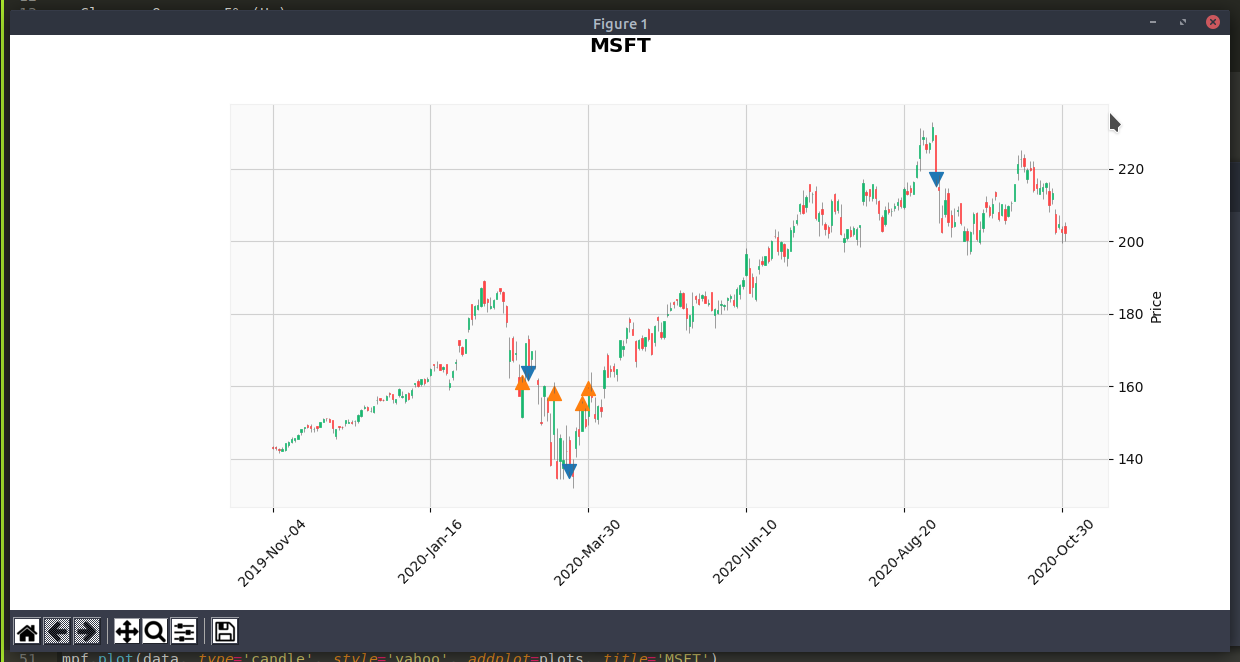# mplfinance Plot Marker on Price Chart

November 3, 2020

We shall plot a marker on the price chart on the following conditions

• Close - Open >= 5% (Up)
• Open - Close >= 5% (Down)
``````import yfinance as yf
import mplfinance as mpf
import numpy as np

yticker = yf.Ticker("MSFT")
data = yticker.history(period="1y") # max, 1y, 3mo, etc

up_markers = []
down_markers = []

for index, row in data.iterrows():
diff = row['Open'] - row['Close']
diff_pct = diff / row['Open']

if diff_pct >= 0.05:
up_markers.append(row['Close'])
down_markers.append(np.nan)
elif diff_pct <= -0.05:
down_markers.append(row['Close'])
up_markers.append(np.nan)
else:
up_markers.append(np.nan)
down_markers.append(np.nan)

return up_markers, down_markers

up_markers, down_markers = detect_intraday_price_diff(data)

up_plot = mpf.make_addplot(up_markers, type='scatter', marker='v', markersize=100, panel=0)
down_plot = mpf.make_addplot(down_markers, type='scatter', marker='^', markersize=100, panel=0)

plots = [up_plot, down_plot]
mpf.plot(data, type='candle', style='yahoo', addplot=plots, title='MSFT')
``````